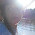## Sunday, June 28, 2015

### 87 96 | SpaceX Rocket Explodes, CNN HeadlinesIt = 9+2 = 11 Is = 9+1 = 10/19 Not = 5+6+2 = 13 Clear = 3+3+5+1+9 = 21 What = 5+8+1+2 = 16 Happened = 8+1+7+7+5+5+5+4 = 42 It Is Not Clear What Happened = 113/122Mainstream = 113Green Screen = 113Dishonest = 113
First, let us decode the headline.
• SpaceX = 1+7+1+3+5+6 = 23/32
• Rocket = 9+6+3+2+5+2 = 27
• Explodes = 5+6+7+3+6+4+5+1 = 37/46
• SpaceX Rocket Explodes = 87/96/105
• This incident comes 87-days before September 23

Interesting numbers indeed, quite Masonic.  Could this be another orchestrated distraction?  A pre Fourth of July display of fireworks?  It comes six days prior, or 144 hours...

Today's date is also interesting.
• 6/28/15 = 6+28+15 = 49
• 6/28/2015 = 6+28+20+15 = 69
• 6/28/2015 = 6+2+8+2+0+1+5 = 24
• 6/28/(2+0+1+5) = 6+28+8 = 42

Also, the names Elon Musk and SpaceX have some slight connections to President Barack Obama.  Perhaps someone is trying to tell us something in a demented way?  Or perhaps this is just a little warm up for July Fourth?Space = 44; X = 24

Also, there is something interesting about 'dragon'.
• Dragon = 4+9+1+7+6+5 = 32

#### 1 comment:

1.Elon Musk has IRON man symbolism...The geeky humanitarian billionaire..with a dumb name...

I bet it is an anagram...for something sinister...

Elon is tool ...posing as the new financial pocket for SOLAR money (it will not be free) electric cars (those will not be free)....and Space EX ploration...( a hoax...which is not free)...and based on a black dragon..and science so false and laughable that I cannot believe that people are not barfing in their beers when they listen to him...